# 机器学习|随机变量（连续型、离散型）+分布函数|10mins入门|概统学习笔记（一）

### 1.随机试验、随机事件、样本空间

• 随机试验：每次出现的可能结果不止一个，且事先不能肯定会出现哪一个结果的试验

• 随机事件：在一次试验中可能发生也可能不发生的事件

• 分类：
• 基本事件：相对于观察目的不可再分解的事件
• 复合事件：两个及以上基本事件合并
• 随机事件的概率:$1 \geq P(A)\geq 0$表示事件A发生概率
• 样本点：随机试验的每个基本结果，记作$e$

• 样本空间：全体样本点的集合，记作$S$e.g 掷骰子

$S=\{i:i=1,2,3,4,5,6\}$

### 2. 随机变量

• 随机变量：定义在样本空间上的实值函数，简称为$r.v.$

• 把随机试验结果数值化

• 随试验结果的不同而取不同的值，在试验之前只知道它可能的取值范围，而不能预先肯定它将取的值

• 由于试验结果的出现具有一定的概率，于是这种实值函数取每个值和每个确定范围内的值也有一定的概率。

• 随机变量通常用大写字母表示$X,Y,Z$$\zeta,\eta$，而随机变量所取的值，通常用小写字母$x,y,z$表示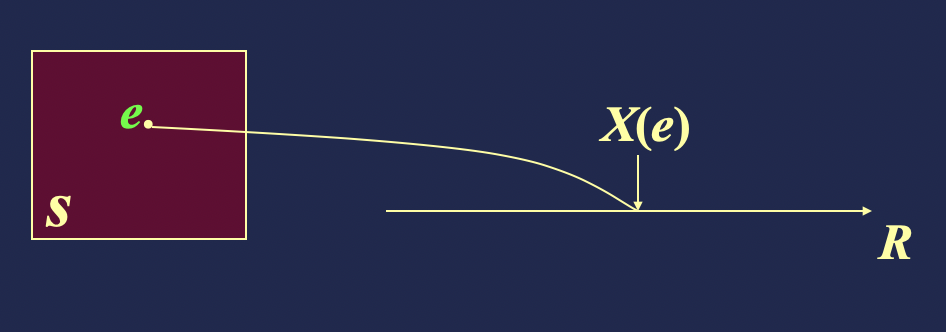• 分类：

• 离散型随机变量：所有取值可以逐个一一列举
• 连续型随机变量：全部可能取值无穷多
• 随机事件与随机变量区别：

1）随机变量包括随机事件

2）随机变量是动态的观点，随机事件是静态的观点，如数学分析中常量与变量的区别

• 离散型随机变量

• 离散型随机变量X的概率函数（或分布律，或概率分布）

$x_k(k=1,2,...)$是离散型随机变量X所取的一切可能值，称
$P(X=x_k)=p_k, k=1,2,...$
其中$p_k(k=1,2,...)$满足：

(1) $p_k \geq 0,k=1,2,...$

(2) $\sum_kp_k=1$

• 离散型随机变量X的概率规律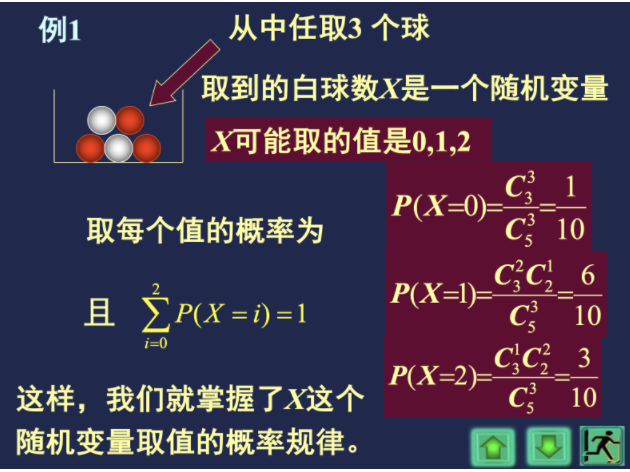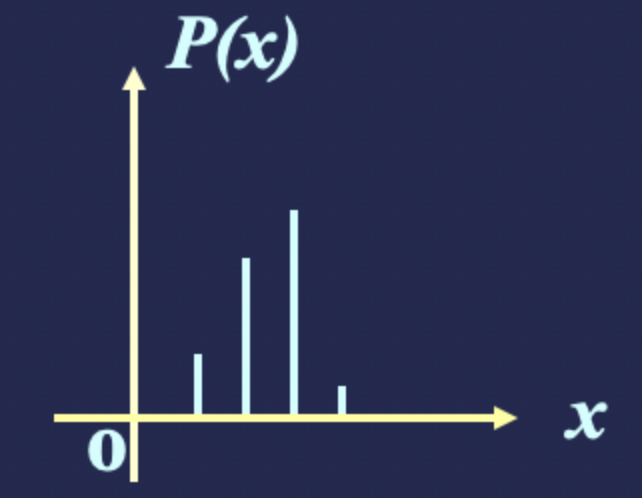• 离散型随机变量由它的概率函数唯一确定
• 连续型随机变量

• 连续型随机变量X的概率密度函数

对于随机变量，如果存在非负可积函数$f(x),x \in (-\infty,+\infty)$,使得对任意$a \leq b$，有
$P(a \leq X \leq b)=\int_a^bf(x)dx$
则称X为连续型$r.v$，称$f(x)$为X的概率密度函数，简称为概率密度。

其中$f(x)$满足：

（1）$f(x)\geq 0$

（2）$\int_{-\infty}^{\infty}f(x)dx=1$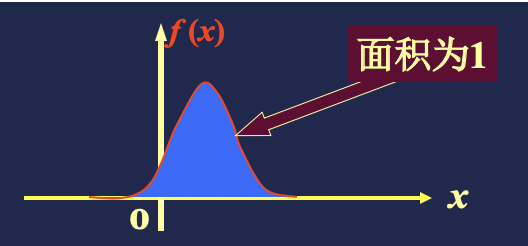• $f(x)$进一步理解：

$x$$f(x)$的连续点，则：
$lim_{\Delta x\to 0}\frac{p(x
故X的密度$f(x)$在x这一点的值，恰好是X落在区间$(x,x+\Delta x]$上的概率与区间长度$\Delta x$之比的极限。这里，如果把概率理解为质量，$f(x)$相当于线密度。

密度函数$f(x)$在某点处$a$的高度，并不反映X取值的概率。但是，这个高度越大，则X取$a$附近的值的概率就越大。这说明某点密度曲线的高度反映了概率集中在该点附近的程度。

• 连续型随机变量取任一指定值的概率为0

即：$P(X=a)=0$$a$为任一指定值

$\because P(x=a)=lim_{\Delta x \to 0}P(a\leq X

• 连续型随机变量唯一被它的密度函数所确定，所以，若已知密度函数，该连续型随机变量的概率规律就得到了全面描述

### 3.分布函数

• 背景：为了对离散型的和连续型的$r.v$以及更广泛类型的$r.v$给出一种统一的描述方法，引进了分布函数的概念。它是一个普通的函数，通过它，我们可以用数学分析的工具来研究随机变量。

• 定义：设X是一个$r.v$，称

$F(x)=P(X\leq x),(-\infty
$X$的分布函数，记作$X$~$F(x)$$F_X(x)$

上式中$X$是随机变量，$x$是参变量。$F(x)$是随机变量$X$取值不大于$x$的概率。

如果将X看作数轴上随机点的坐标，那么分布函数$F(x)$的值就表示X落在区间$(-\infty,x]$的概率。对任意实数$x_1 ，随机点落在区间$(x_1,x_2]$的概率为：
$P\{x_1
因此，只要知道了随机变量$X$的分布函数，它的统计特性就可以得到全面的描述

• 分布函数的性质：

（1）$F(x)$非降，即若$x_1，则$F(x_1)\leq F(x_2)$

（2）$F(-\infty)=lim_{x\to -\infty}F(x)=0$

$F(+\infty)=lim_{x\to +\infty}F(x)=1$

（3）$F(x)$右连续，即$lim_{x\to x_0^+}F(x)=F(x_0)$

• 离散型随机变量的分布函数

设离散型随机变量$X$的概率函数是
$P\{X=x_k\}=p_k, \quad k=1,2,3,...$

$F(x)=P(X\leq x)=\sum_{x_k\leq x}p_k$
由于$F(x)$$X\leq x$的诸值$x_k$的概率之和，故又称$F(x)$为累积概率函数

• 连续型随机变量的分布函数

$X$是连续型随机变量，$X$~$f(x)$，则
$F(x)=P(X\leq x)=\int_{-\infty}^xf(t)dt$
即分布函数是密度函数的可变上限不定积分

由上式可得，在$f(x)$的连续点处，有
$\frac{dF(x)}{dx}=f(x)$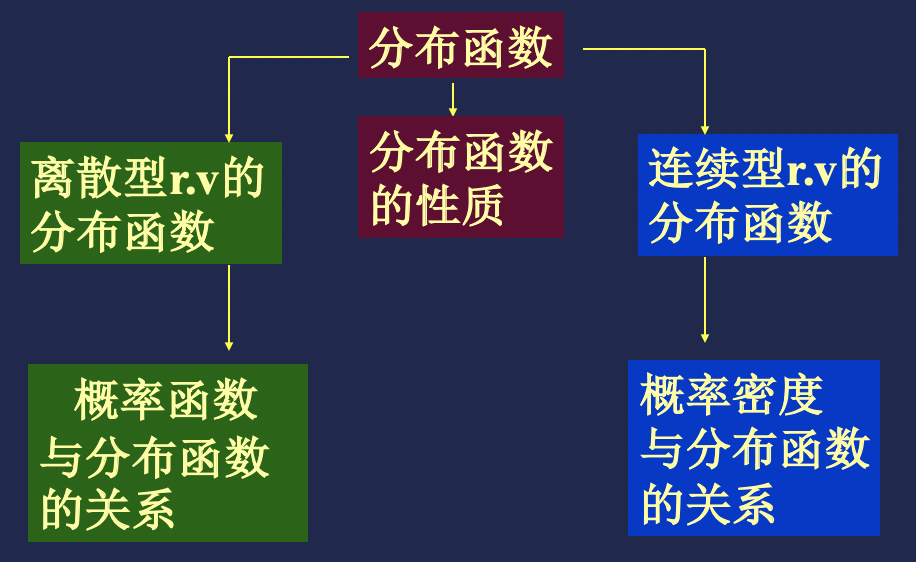©️2019 CSDN 皮肤主题: 数字20 设计师: CSDN官方博客